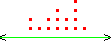www.batmath.it

A Glossary of Mathematical Terms - L

Lattice points

Points in the coordinate plane with integer coordinates.

Least common multiple (L.C.M.)

he least common multiple of two or more natural numbers is the smallest counting number that is a multiple of each of the given numbers.

Like terms

In an expression, tyerms that have the same variables raised to the same exponents (so that they can be summed up).

Line

A straight path that proceeds inifinitely in two directions.

Line graph

A kind of plot for data, when dots are joined by segments. Also Broken line graph.

Line of symmetry

A line that divides a figure into to parts, each a mirror of the other.

Line plotA display of data along the real line, with some symbol indicating the frequency or tally of information about a single category.

Linear equation

A first degree euqation.

Linear pair (of angles)

Two adjacent angles whose sum is 180°. (In Italian two angles with this property are simply "adiacenti").

Linear term of an equation

The term with the variable at the first degree.

Locus (pl. loci)

A set of points (in the line, in the plane or in the space) that satisfy a given condition.

Logarithmic function

A function of the form y = loga(x).

Lowest terms

See Fraction.

first published on september 01 2003 - last updated on september 01 2003